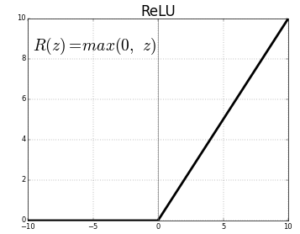# ReLU function

A ReLU function, meaning rectified linear unit function, is an activation function used in neural networks to define the output of a node given a set of inputs. It uses the rectifier, a function that can be defined as the positive part of its argument, or with the formula:The graph representing this function is as follows, from R to ]0;+∞[Introduced in the 2010s the ReLU function has become the most used activation function in neural networks, replacing the Sigmoid function which was traditionally used before.

Read more on the rectifier and the ReLU function on Wikipedia.

« Back to Glossary Index